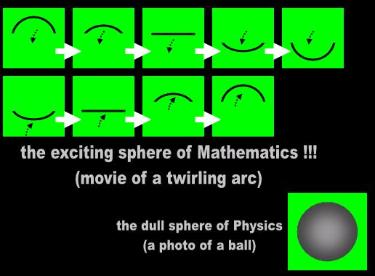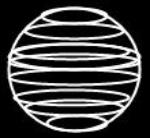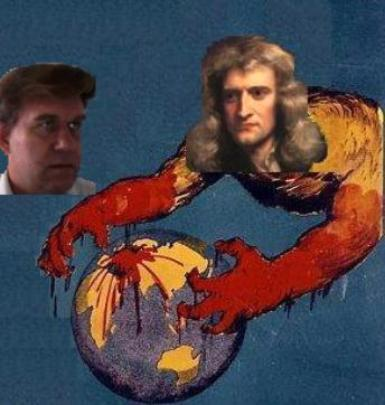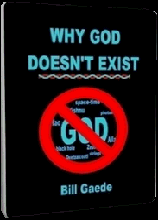A mathematician sculpts solids point by point
 Adapted for the Internet from:Why God Doesn't Exist

1.0   Geometric figure or volume?

The mathematicians are ultimately not concerned with genuine geometric figures. They have no interest in circles, triangles
and squares per se. Mathematicians are concerned with relations. They are interested in parameters such as area, diameter,
length of a side, height of a polygon, radius, etc. A square is otherwise a stale object for them. It has no purpose or meaning
to a mathematician if he cannot study its internal relations or use it for something.

It was the internal relations of the basic planes and solids that captured the imagination and fascination of the Greeks. When
Plato and Euclid talk about a sphere, they really have in mind a volume.  They want to figure out, for example, how to
calculate the volume a sphere displaces. This is the type of activity that gives a mathematician a rush. The Greeks had
otherwise no use for the square or the circle as mere objects. Likewise, the early mathematicians defined solids specifically
for the purposes of establishing relations. A cube and a sphere have absolutely no importance to a geometer strictly as
figures.

Hence, the mathematicians developed definitions useful to their line of work and which are unrelated to Physics or to the
real physical world. This explains why they have never found a need to define the word plane correctly. They can perform
their mathematical operations even with faulty definitions and conceptualizations because the mathematicians never have
to produce the goose that lays the golden eggs. A mathematician produces nothing tangible in his entire existence!

One of the earliest purposes for which the mathematicians found planes useful was to calculate volumes. The
mathematician takes a square, scans it through space, and tells you that you end up with a cube. However, when you
attempt to reproduce his experiment in your basement, you always end up with something else. First, you realize that you
cannot scan a square because in Physics a square is not a stand-alone figure. But assuming you could, you still don't end
up with a cube. You end up with a stack of squares! Is a pile of paper equivalent to a box? The mathematicians really have
to be kidding!

What the mathematicians are doing in effect is 'scanning' an area and 'constructing' a volume. The side of this 'cube' is not
a surface you can stand on, but rather a distance-traveled, a movie of the alleged area at different locations.

Let's put all of this in mathematical terms so that the mathematicians may also understand what it is that they are doing.
The mathematician erroneously believes and misinforms you that he took a square, multiplied it by a length, and ended up
with a cube:

cube = square * length

However, the units he attaches to his result makes you suspect that he lost a screw somewhere in his head:

14 cubic meters or 14 m³

What the stupid fool actually did was scan an area and build a volume:

volume = area * distance-traveled

We are not talking about cubes or squares, which means that we are not yet talking about Geometry. We are talking about
building a volume by scanning an area. A cube is a static geometric figure. A volume is a dynamic concept we build by
expansion or shrinkage. A rubber ducky is a physical object and may be said to belong to Geometry. The amount of water
it displaces is a volume. A volume has nothing to do with Geometry and all to do with Math. Only the idiots of Mathematics
confuse one with the other.

It is using 'constructions' that the mathematical morons arrive at their dynamic 'geometric figures' with which they live today.
All of the 'figures' of Mathematical Physics are conveniently both static and dynamic. The mathematician points to a cube
and tells you that it is a geometric figure. We have no problem with this. He tells you next that he 'constructed' this cube by
scanning a plane or integrating dots. Now we do have a problem. This last claim amounts to a volume. A volume is not the
same 'thing' as a cube. A volume is a dynamic concept. A cube is a static object.

This duality comes in very handy to a mathematician, especially when he attempts to give a physical interpretation to an
equation. He has unwittingly established the foundations that enable him to jump back and forth between architecture and
motion.

2.0   Euclid's 'sphere': Twirling the semicircle

One of the most popular 'constructions' of Mathematical Physics is the ever present sphere. There are several ways in which
the mathematicians 'construct' the volume of a sphere. Euclid did it the old-fashioned way. He took a semicircle and twirled it
around an axis (Fig. 1):

When a semicircle with fixed diameter is carried round and restored again to the same
position from which it began to be moved, the figure so comprehended is a sphere.”
(Bk. 11, Def. 14)  

a sphere may be generated by revolving a semicircle through 360º about its diameter
as the axis.”  

A surface of revolution is a surface generated by rotating a two-dimensional curve

Unfortunately, what Euclid and his contemporary disciples are proposing ends up being nothing like a sphere. Euclid is
talking about a movie rather than about a photograph. Do the following experiment at home, but always under the
supervision of a trained mathematician. Take a clothes-hanger and mold it so that it looks like a semicircle. Twirl it around
'its diameter as the axis.' Did you end up with a sphere? A sphere looks more or less like a bowling ball or like a globe. If
you ended up with a warped clothes hanger, you now should know the difference between Math and Phyz! The
mathematical morons are not talking about a sphere or about dimensions or about a surface of anything. Again, they are
explaining the technique that enabled them to come up with a volume.

3.0   The 'sphere' of Modern Math: An empty shell made of locations

Euclid's modern day disciples have come up with better mousetraps. They don't always twirl semicircles. Sometimes they
open up an umbrella of points (Fig. 2):

" a sphere is the set of all points in three-dimensional space (R3) which are at
distance r from a fixed point" 

Other times they have a single point (meaning location) orbiting a center.

" The sphere itself now consists of all points satisfying the equation: x² + y² + z² = r² " 

The equation definition of a sphere invariably requires that you to construct one point at a time.

And yet other times, people visualize the sphere as a series of piled up rings (Fig. 3):

" A sphere is a sequence of concentric circles laying top to bottom on each other" 52b 

So one of the questions that rational people raise is whether a sphere is supposed to be made of parts or of a single piece.
Is a sphere a segmented or a continuous object? Will a sphere that is made of smaller components be ideally smooth? For
instance, pursuant to these specifications, Wolfram illustrates a disco ball, which Wikipedia specifically qualifies as being 'a
roughly spherical object'. Is this what a sphere is? A million-sided polyhedron? Is a sphere something we construct over time
or is it something you point to and visualize in a single stare? Are we supposed to imagine a statue or a statue in the making?
 Fig. 1   Sphere: The movieTwirling the semicircleI could not visualize you in one go, so I had to construct you piece by piece. When God does not bless us with the ability to conceptualize, we must build our own dreams and visions.
 A relativist ‘constructs’ a sphere by spinning a curved clothes hanger. When the mathematician is finished, she ends up with a curved clothes hanger and not with a sphere. Relativists confuse a movie of how a sphere was built (dynamic) with a sculpture (static). The sphere of the mathematicians belongs neither in Geometry nor in Physics.Fig. 2   Sphere: The photograph           equidistant dots (or locations)Nevertheless, notice that none of these proposals results in a bowling ball. The mathematicians are not talking about a
solid. They are talking about a 'hollow.' The sphere of Mathematics is an empty balloon. But it is not even a balloon. The
rubber of this alleged balloon is not made of matter. The entire surface is merely a collection of locations. There is
absolutely nothing occupying each of those locations. Each 'point' comprising the 'surface' of the mathematical sphere
is an abstract ordered triple (x, y, z). When you peel mathematical onion to the core, you always end up with zip! There is
nothing you can sink your teeth into. The sphere of Mathematics is not a physical object because it has no shape.
According to the specifications, there is nothing in front of you.

The mathematician says 'So what? We deal with concepts. This enables us to communicate ideas without being distracted
by architecture.'

Aaahh! But then you cannot extrapolate your nonsense to Physics. If you want to cross the line into Physics, you must
absolutely leave your abstract garbage behind. You cannot use your sphere in our discipline. It is okay if you want to
brainstorm some nonsense in your petty world of make-believe. We have no problem with that. But if you want to theorize
about something in the real world, you now will be required to use the sphere of Physics: the bowling ball. And if your
theory cannot work with the solid ball we put in front of you, whatever you concocted in the fantasy world of Math is
irrelevant. There is no such sphere as the abstract mathematical sphere anywhere in the Universe! Yet this is exactly the
sphere which the idiots of Mathematics use to reach ridiculous syntheses such as the black hole. The mathematical
moron does not compress a ball such as a star. He shrinks its volume. He compresses its  mass. That's why the stupid
idiot doesn't see any problem with shrinking this volume to 0 and calling the result a singularity, insinuating in the
process that the singularity has something to do with Physics. The idiot of Mathematics has no street smartness, no n
atural curiosity to question whether such hogwash has anything to do with reality. He accepts the conclusions merely
because the results follow from his equations.

4.0   Conclusions

The fundamental problem with the 'construction' techniques used by the mathematicians is that they don't produce
geometric figures. Instead, they result in volumes. The mathematician is not interested in a sphere. The mathematician
is at best interested in the volume displaced by the sphere. The second problem with 'constructions' is that you cannot
see them in a single stare. You must sit through an eternal movie of a sculptor chiseling away one point at a time. The
dynamic 'figures' of Mathematics are not stand-alone statues you can touch in the lab. They are abstractions you must
watch at the movies. Since the sphere is alleged to be made of 'infinite points,' this movie does not have a very happy
ending.  The 'solids' of Mathematics are perpetually under construction. But even if we accept the 'equidistant points'
as a photograph, this version contrasts with the dynamic twirling semicircle that Euclid had in mind. These version
conduce to different physical interpretations and really constitute an attempt to embody both static and dynamic features
into one 'geometric figure.' This leads me to conclude that we have to revise any inferences drawn from the sphere of
Mathematics in the last 4000 years.

The sad history of the 'solid' known as the sphere has been a gradual disappearance of matter: from dense bowling ball
to hollow balloon and from a shell to a little dot (that is really an abstract location) still going around in an incessant
attempt to enclose a volume of nothing. The mathematicians no longer point or even describe WHAT a sphere IS. They
explain how they are building it!
 Fig. 3   Sphere: The photograph         piled up ringsIf you want the sphere to be smoother, all you have to do in Mathematical Physics is dig your fingernails in it like this to generate more points.